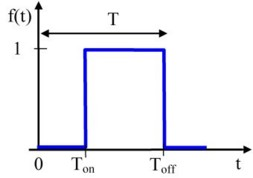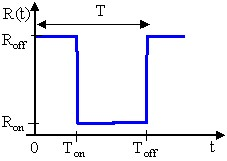# Semi-conductor components: command mode

## Command signal

The command mode is a dependence f(t) that can be a function:

• defined by the user (formula)
• crenel

## Crenel function

The crenel function is a function that defines a crenel. It is presented in the figure below.

 T is the period Ton is the turn on time Toff is the turn off time## Switch example

The Switch component is a semi-conductor component controlled at the opening and at the closing, independent of the condition at its terminals.

An example of the R(t) characteristic of a switch is presented in the figure below.

 T is the period Ton is the turn on time Toff is the turn off time Ron is the on state resistance Roff is the off state resistance